## Setup

You will need these packages. Must use the development version of `hydromad`, requires a working compiler (RTools on Windows).

``````library(aqp)
library(soilDB)
library(sharpshootR)

# special installation instructions:

## Simple Example

Simulate monthly water balances for 50cm of sandy loam vs. 50cm of silt loam soil material in a xeric/thermic climate.

``````data("ROSETTA.centroids")

idx <- which(ROSETTA.centroids\$texture %in% c('sandy loam', 'silt loam'))
vars <- c('texture', 'pwp', 'fc', 'sat', 'awc')

knitr::kable(
ROSETTA.centroids[idx, vars],
row.names = FALSE
)``````
texture pwp fc sat awc
sandy loam 0.0624426 0.167354 0.3870001 0.1049114
silt loam 0.0858854 0.293965 0.4390000 0.2080796
``````# near Sonora, CA
# thermic / xeric climate
# units are mm
PET <- c(0, 0, 5,80, 90, 120, 130, 140, 110, 90, 20, 5)
PPT <- c(0, 150, 200, 120, 20, 0, 0, 0, 10, 20, 30, 60)

# 50cm of soil material -> convert to mm
thick <- 50 * 10

# total water storage for sandy loam texture soil material
AWC.sl <- round(0.1049114 * thick)

# total water storage for silt loam texture soil material
AWC.sil <- round(0.2080796 * thick)

# simulate water balances:
# * run for 5 cycles
# * start in January
# * keep only the last cycle
wb.sl <- monthlyWB(AWC.sl, PPT, PET, S_init = 0, starting_month = 1, rep = 5, keep_last = TRUE)
wb.sil <- monthlyWB(AWC.sil, PPT, PET, S_init = 0, starting_month = 1, rep = 5, keep_last = TRUE)``````

All values are mm. U: surplus, S: soil water storage, ET: actual ET, D: deficit.

``kable(wb.sil, digits = 2, row.names = FALSE)``
PPT PET U S ET D month mo
0 0 0.00 82.25 0.00 0.00 1 Jan
150 0 128.25 104.00 0.00 0.00 2 Feb
200 5 195.00 104.00 5.00 0.00 3 Mar
120 80 40.00 104.00 80.00 0.00 4 Apr
20 90 0.00 34.00 90.00 0.00 5 May
0 120 0.00 0.00 34.00 -86.00 6 Jun
0 130 0.00 0.00 0.00 -130.00 7 Jul
0 140 0.00 0.00 0.00 -140.00 8 Aug
10 110 0.00 0.00 10.00 -100.00 9 Sep
20 90 0.00 2.69 17.31 -72.69 10 Oct
30 20 0.00 26.41 6.29 -13.71 11 Nov
60 5 0.00 82.25 4.15 -0.85 12 Dec
``````# compare
par(mar=c(4,4,4,1), bg = 'white', mfcol = c(1, 2))
plotWB(WB = wb.sl, legend.cex = 0.75)
title('50cm of sandy loam texture soil material', line = 3)

plotWB(WB = wb.sil, legend.cex = 0.75)
title('50cm of silt loam texture soil material', line = 3)``````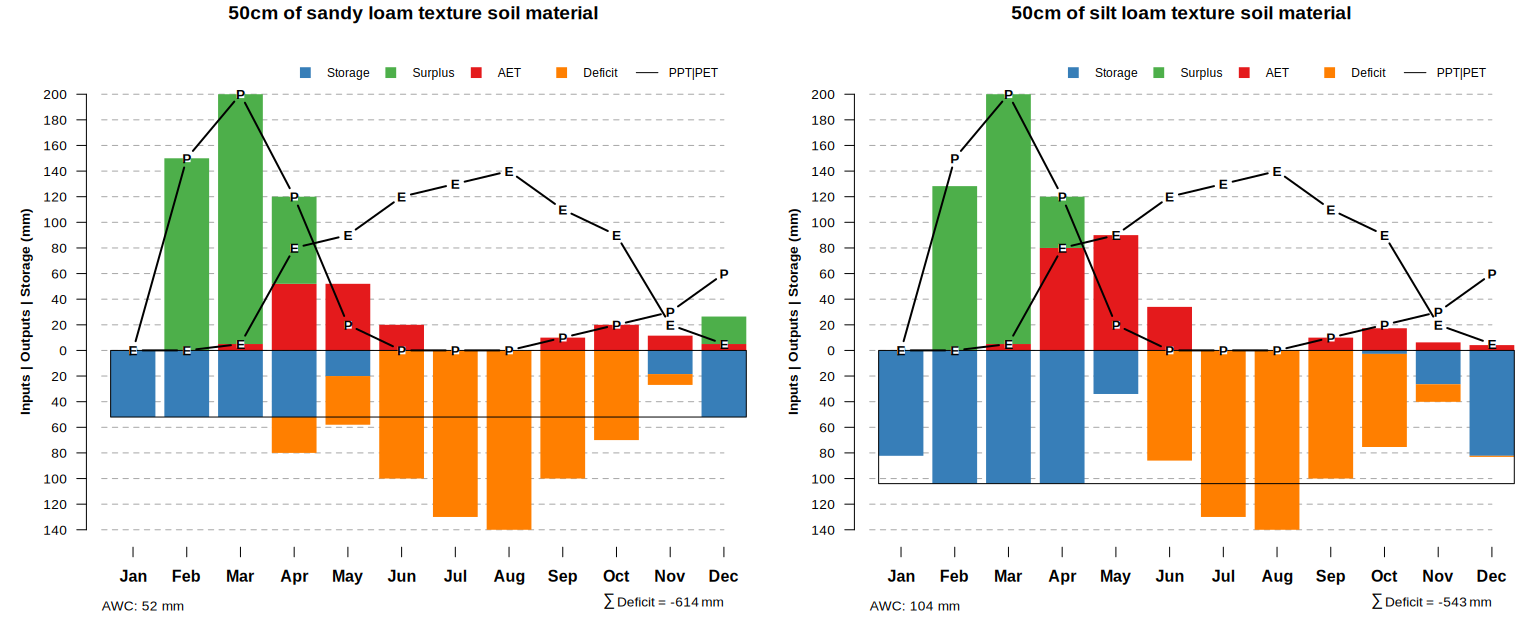``````# compare
par(mar=c(4,4,4,1), bg = 'white', mfcol = c(1, 2))
plotWB_lines(WB = wb.sl)
title('50cm of sandy loam texture soil material', line = 3)

plotWB_lines(WB = wb.sil)
title('50cm of silt loam texture soil material', line = 3)``````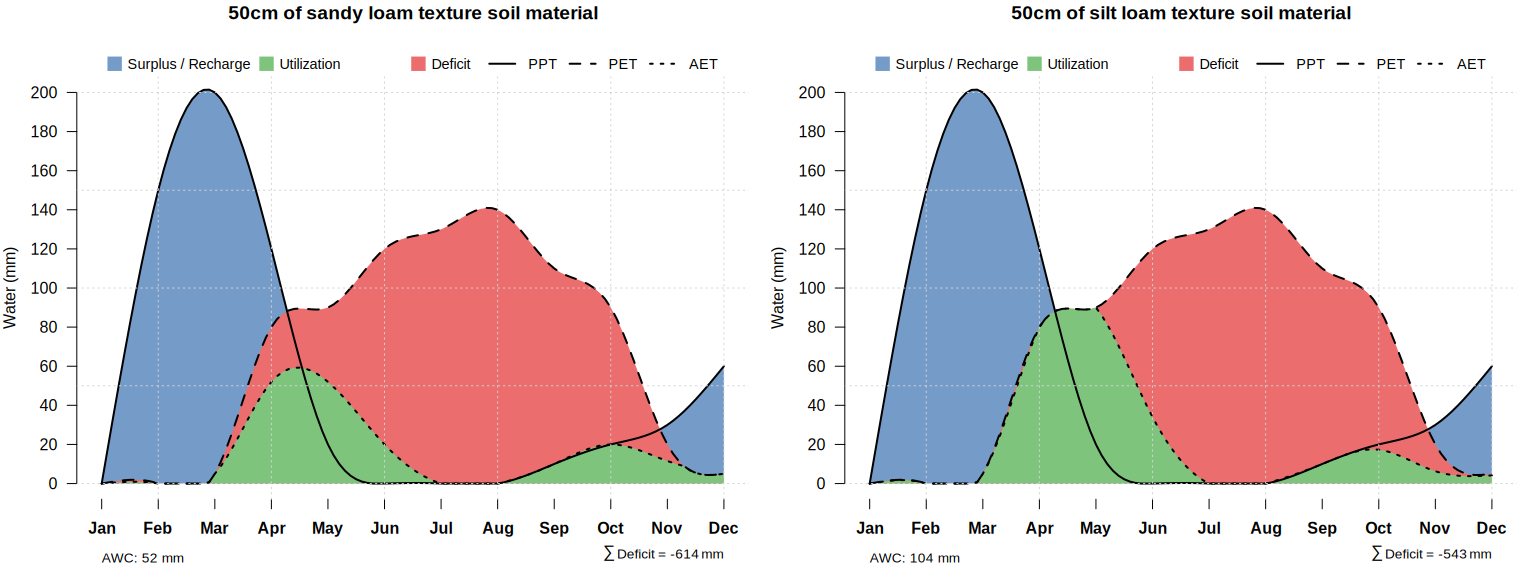## Real Data

Series-level summaries of climate provided by `fetchOSD()`.

AWC estimated from SSURGO data, via SDA.

``````s <- 'Amador'
x <- fetchOSD(s, extended = TRUE)

# get representative, profile-total AWC from SSURGO
sql <- sprintf("
SELECT chorizon.cokey AS cokey,
SUM(awc_r * (hzdepb_r - hzdept_r)) AS ws
FROM
legend JOIN mapunit ON legend.lkey = mapunit.lkey
JOIN component ON mapunit.mukey = component.mukey
JOIN chorizon ON component.cokey = chorizon.cokey
WHERE compname = '%s'
AND areasymbol != 'US'
GROUP BY chorizon.cokey;", s
)

# get via SDA
res <- SDA_query(sql)

# median AWC in mm
# over all components correlated to named series
AWC <- round(median(res\$ws, na.rm = TRUE) * 10)

# monthly climate data from series summary
PPT <- x\$climate.monthly\$q50[x\$climate.monthly\$variable == 'Precipitation (mm)']
PET <- x\$climate.monthly\$q50[x\$climate.monthly\$variable == 'Potential ET (mm)']``````

Water year.

``````# tighter margins
par(mar=c(4,4,2,1), bg = 'white')

# water year
# last iteration
x.wb <- monthlyWB(AWC, PPT, PET, S_init = 0, starting_month = 9, rep = 3, keep_last = TRUE)
plotWB(WB = x.wb)``````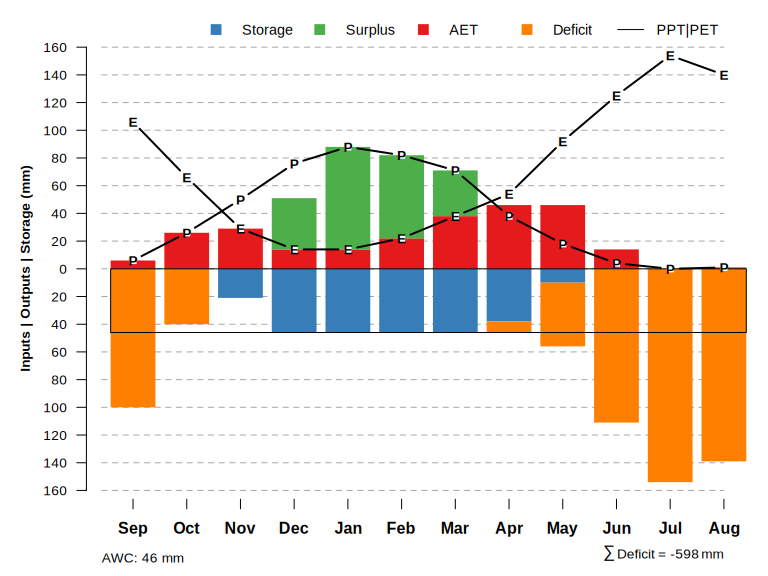``````# convert total ETa into inches
sum(x.wb\$ET) * 0.03937``````
``##  10.07872``

Calendar year.

``````# tighter margins
par(mar=c(4,4,2,1), bg = 'white')

# calendar year
# last iteration
x.wb <- monthlyWB(AWC, PPT, PET, S_init = 0, starting_month = 1, rep = 3, keep_last = TRUE)
plotWB(WB = x.wb)``````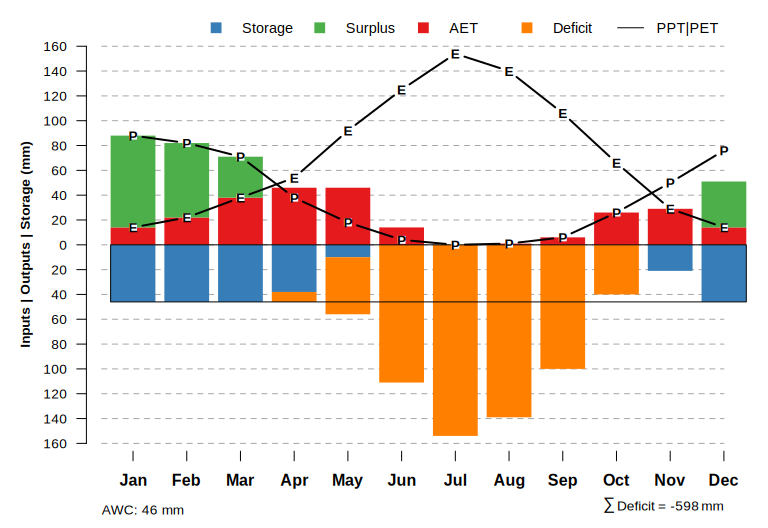### Bar vs. Line Graph

``````s <- 'auburn'
x <- fetchOSD(s, extended = TRUE)

# get representative, profile-total AWC from SSURGO
sql <- sprintf("SELECT chorizon.cokey AS cokey,
SUM(awc_r * (hzdepb_r - hzdept_r)) AS ws
FROM
legend JOIN mapunit ON legend.lkey = mapunit.lkey
JOIN component ON mapunit.mukey = component.mukey
JOIN chorizon ON component.cokey = chorizon.cokey
WHERE compname = '%s'
AND areasymbol != 'US'
GROUP BY chorizon.cokey;", s)

# get via SDA
res <- SDA_query(sql)

# median AWC in mm
# over all components correlated to named series
AWC <- round(median(res\$ws, na.rm = TRUE) * 10)

# monthly climate data from series summary
PPT <- x\$climate.monthly\$q50[x\$climate.monthly\$variable == 'Precipitation (mm)']
PET <- x\$climate.monthly\$q50[x\$climate.monthly\$variable == 'Potential ET (mm)']

# 3 warm-up cycles
# keep last iteration
# calendar year
x.wb <- monthlyWB(AWC, PPT, PET, S_init = 0, starting_month = 1, rep = 3, keep_last = TRUE)

# tighter margins
par(mar=c(4,4,3,1), bg = 'white', mfcol = c(1, 2))

plotWB(x.wb)
title(sprintf('Monthly Water Balance: %s Series', toupper(s)), line = 2)

plotWB_lines(x.wb)
title(sprintf('Monthly Water Balance: %s Series', toupper(s)), line = 2)``````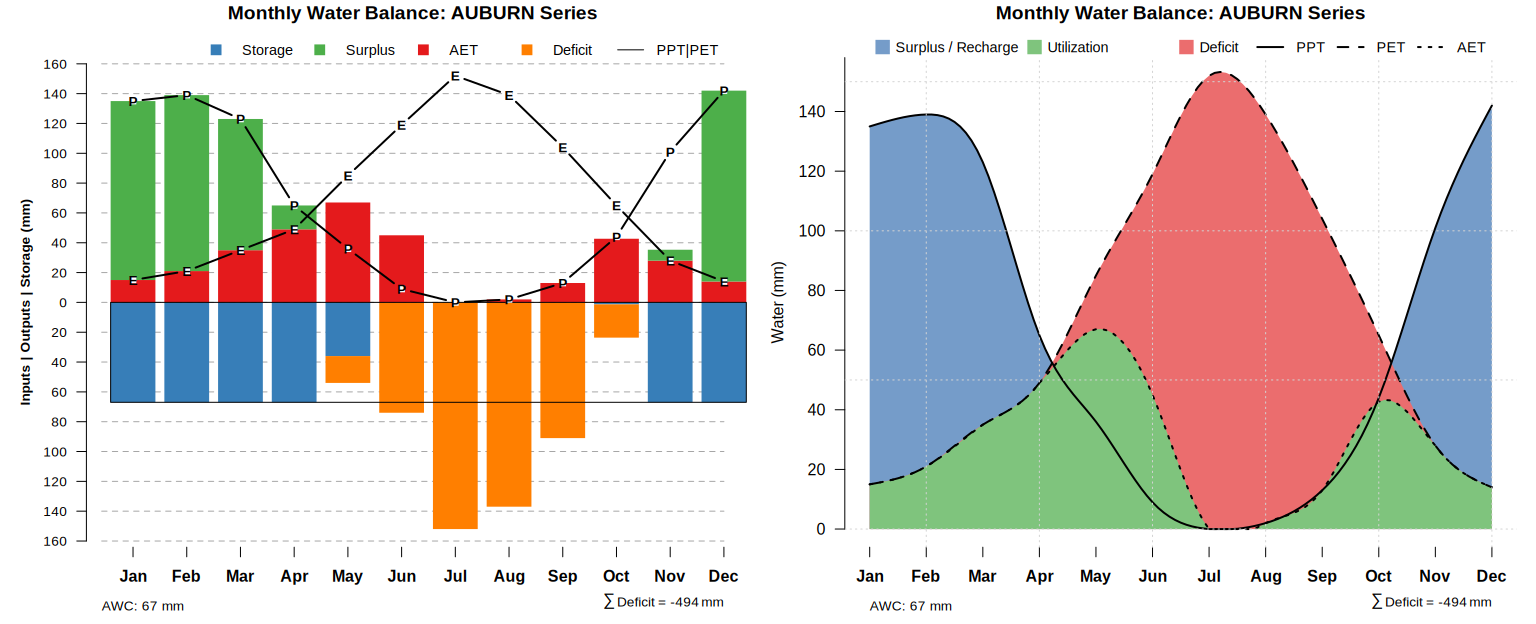## Comparisons

Abstract into a function, and use to compare multiple soils / climatic parameters. A more efficient approach would load all of the data then iterate over chunks.

``````# x: soil series name
compareMonthlyWB <- function(x) {

# get climate data
osd <- fetchOSD(x, extended = TRUE)

# get representative, profile-total AWC from SSURGO
sql <- sprintf("
SELECT chorizon.cokey AS cokey,
SUM(awc_r * (hzdepb_r - hzdept_r)) AS ws
FROM
legend JOIN mapunit ON legend.lkey = mapunit.lkey
JOIN component ON mapunit.mukey = component.mukey
JOIN chorizon ON component.cokey = chorizon.cokey
WHERE compn``````